# Ten Equations That Changed The World

The pulse of the earth is mathematics.

The brightest minds in history have used mathematics to lay the foundation for how we measure and understand our universe. Time and time again, we have proved that it only takes one simple formula to alter the course of humanity. Here are ten equations that did just that.

### 1.  Isaac Newton’s Law Of Universal Gravitation: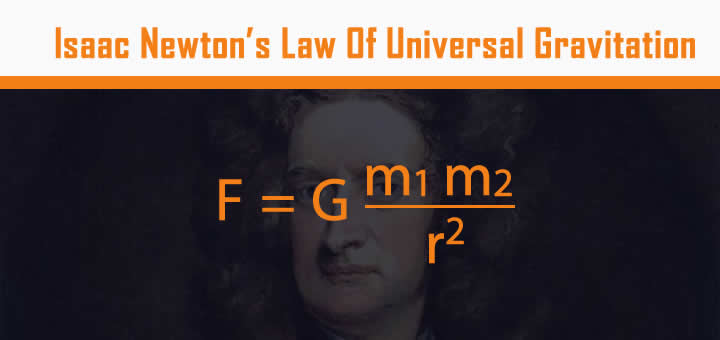Newton’s law explains why the planets move the way they do, and how gravity works, both on Earth and throughout the Universe. First published in The Principia in July 1687, the Law of Universal Gravitation was the defacto reference equation for nearly 200 years until Einstein’s Theory of General Relativity replaced it.

### 2. Albert Einstein’s Theory Of Relativity: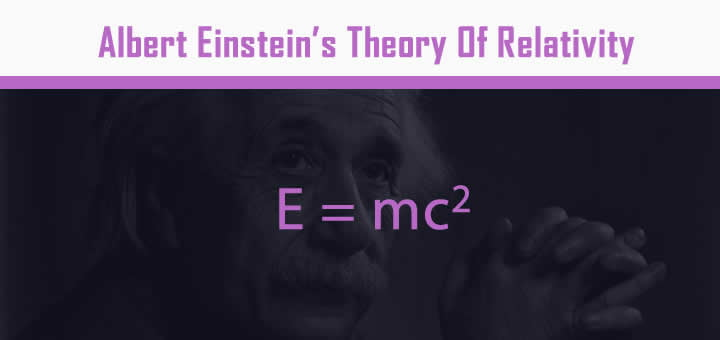Einstein’s most famous undertaking is the generally accepted theory on the relationship between space and time. First Proposed in 1905, the Theory of Relativity has both radically altered the course of physics, and deepened our knowledge of the Universe’s past, present, and future.

### 3. The Pythagorean Theorem:This ancient theorem — first recorded circa 570 – 495 BC — is a fundamental principle in Euclidean Geometry, and the basis for the definition of distance between two points. Pythagoras’ theorem also describes the relationship between the sides of a right triangle on a flat plane.

### 4. Maxwell’S Equations: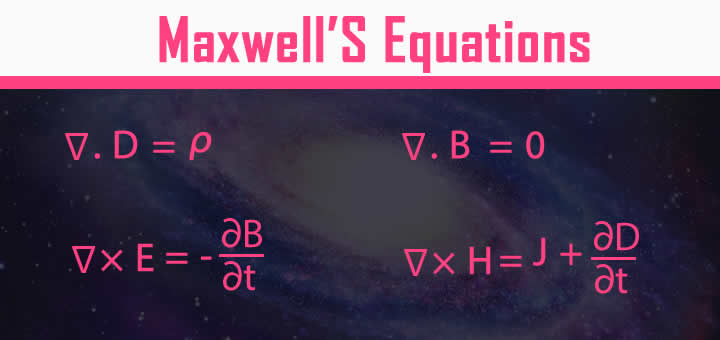James Clerk Maxwell’s set of equations describe how electric and magnetic fields are generated and altered, both by each other and by charges and currents. First published between 1861 and 1862, they are to classical electromagnetism what Newton, laws of motion and universal gravitation are to classical mechanics.

### 5. The Second Law Of Thermodynamics:Rudolf Clausius’ law states that energy , always flows from higher concentration to , lower concentrations. It also states that whenever energy changes or moves, it becomes less useful. Formulated in 1865, it has led to the development of technologies like internal combustion engines, cryogenics, and electricity generation.

### 6. Logarithms:Logarithms were introduced by John Napier in the early 17th century as a way to simplify calculations. They answer the question, “How many of X number do we multiply to get Y number?”. Logarithms were adopted by early navigators, scientists and engineers. Today, scientific calculators and digital computers do the work for us.

### 7. Schrödinger Equation: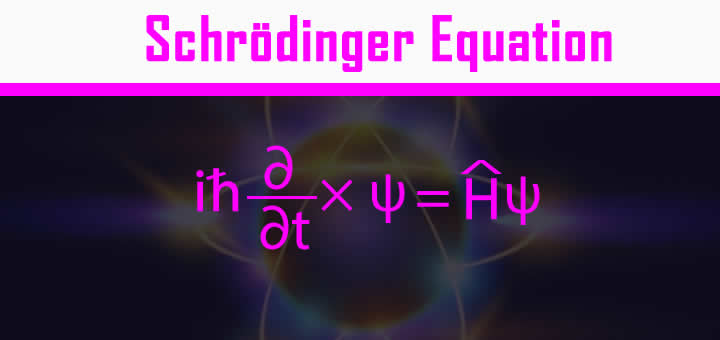This equation describes how the quantum state of a quantum system changes with time. Developed by Austrian physicist Erwin Schrödinger in 1926, it governs the behavior of atoms and subatomic particles in quantum mechanics. Schrödinger’s Equation paved the way for nuclear power, microchips, electron microscopes, and quantum computing.

### 8. Chaos Theory: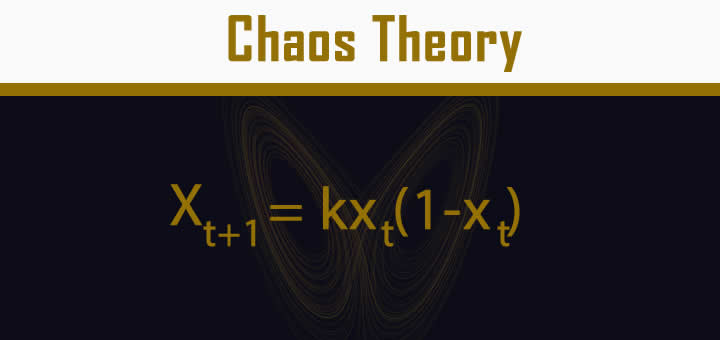Chaos Theory is a branch of mathematics that studies complex systems whose behavior is extremely sensitive to slight changes in conditions. In essence. it shows how small alterations can lead to consequences of much greater scale. Chaos Theory has applications just about everywhere — meteorology, sociology, physics, computer science, engineering, economics, biology, and philosophy.

### 9. Calculus: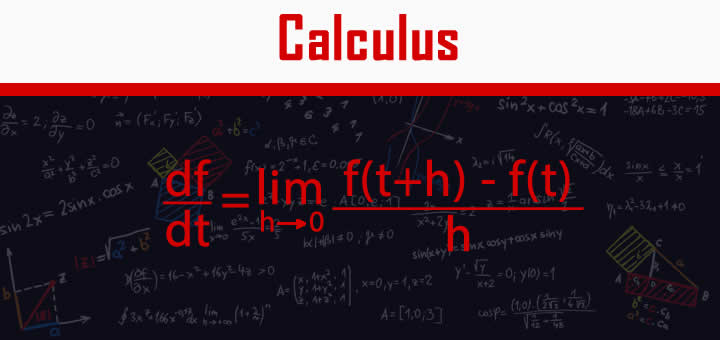The calculation shown is the definition of the derivative in differential calculus, one of calculus’ two major branches. The derivative measures the rate at which a quantity is changing — if you are walking 2 km an hour, then you will change your position by 2 km every hour. In the 1600s, Newton used calculus to develop his laws of motion and gravitation.

### 10. Information Theory: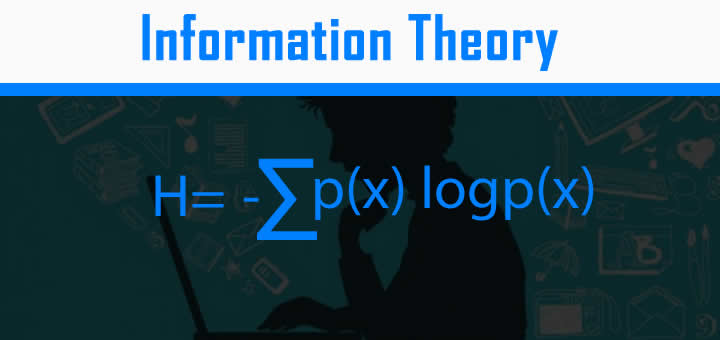Information theory is a branch of mathematics that studies the coding of information in the form of sequences of symbols, and the speed at which that information can be transmitted. Applications of topics within information theory include data compression and channel coding. Research in the field was also instrumental in the development of the internet and mobile phones.

This site uses Akismet to reduce spam. Learn how your comment data is processed.

This website uses cookies to improve your experience. We'll assume you're ok with this, but you can opt-out if you wish. Accept Read More